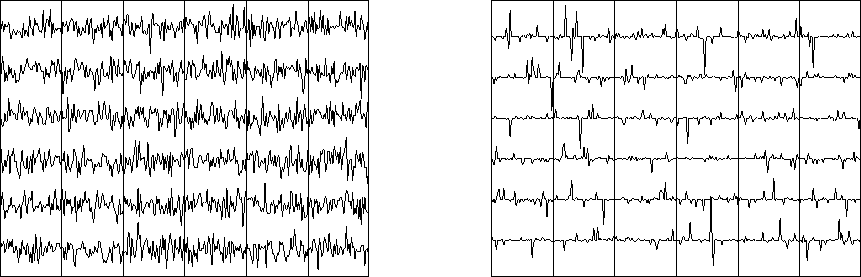Next: Random numbers into a Up: SYNTHETIC DATA FROM FILTERED Previous: SYNTHETIC DATA FROM FILTERED

## Gaussian signals versus sparse signals

Most theoretical work is based on random numbers from a Gaussian probability function. The basic theoretical model is that at every time point a Gaussian random number is produced. In real life we do observe such signals, but we also observe signals with less frequent noise bursts. Such signals, called ``sparse signals'' or ``bursty signals,''

can be modeled in many ways, two of which are (1) that many points can have zero value (or a value that is smaller than expected from a Gaussian); and (2) that the Gaussian probability function describes the many smaller values, but some larger values also occur from time to time.

It turns out that the Gaussian probability function generates more cryptic signals than any other probability function. It also turns out that theory is best developed for the Gaussian case. Thus, Gaussian theory, which is the most pessimistic, tends to be applied to both Gaussian and sparser data. Sparse signals derive from diverse models, and usually there is not enough information to establish a convincing model. In practical work, ``non-Gaussian'' generally means ``sparser than Gaussian.'' Figure 4 illustrates random signals from a Gaussian probability function and a sparser signal made by cubing the random numbers that emerge from a Gaussian random-number generator.spikes
Figure 4
Left are random numbers from a Gaussian probability function. (The random numbers are connected by lines.) Right, the random numbers are cubed, making a signal in which large spikes are sparser.Next: Random numbers into a Up: SYNTHETIC DATA FROM FILTERED Previous: SYNTHETIC DATA FROM FILTERED
Stanford Exploration Project
10/21/1998